# Human Animal and Machine Learning Vasile Rus http

• Slides: 37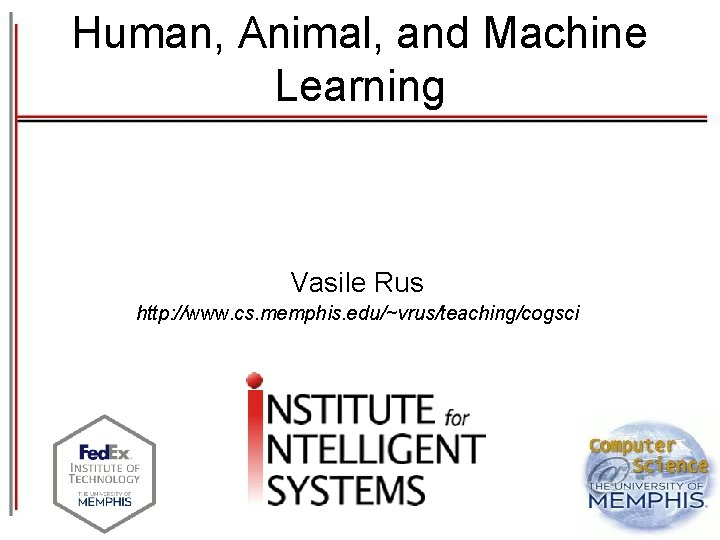Human, Animal, and Machine Learning Vasile Rus http: //www. cs. memphis. edu/~vrus/teaching/cogsci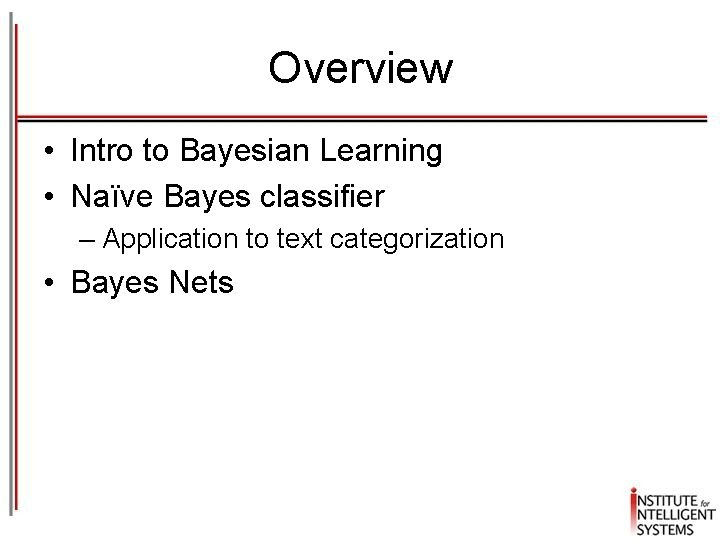Overview • Intro to Bayesian Learning • Naïve Bayes classifier – Application to text categorization • Bayes Nets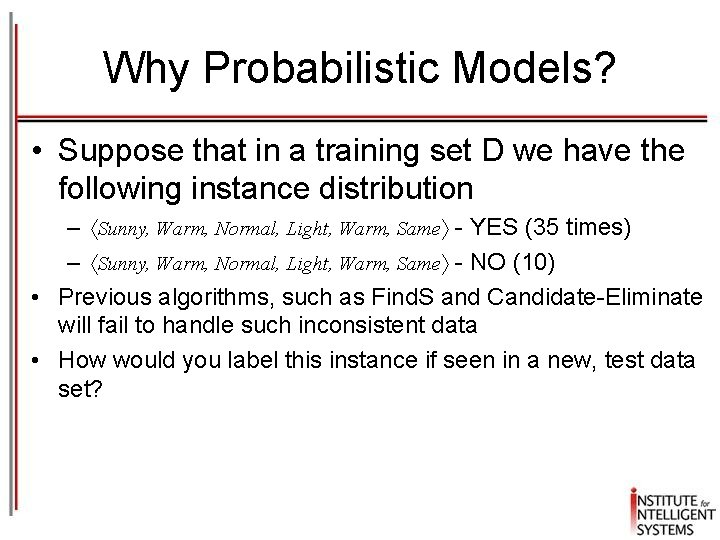Why Probabilistic Models? • Suppose that in a training set D we have the following instance distribution – Sunny, Warm, Normal, Light, Warm, Same - YES (35 times) – Sunny, Warm, Normal, Light, Warm, Same - NO (10) • Previous algorithms, such as Find. S and Candidate-Eliminate will fail to handle such inconsistent data • How would you label this instance if seen in a new, test data set?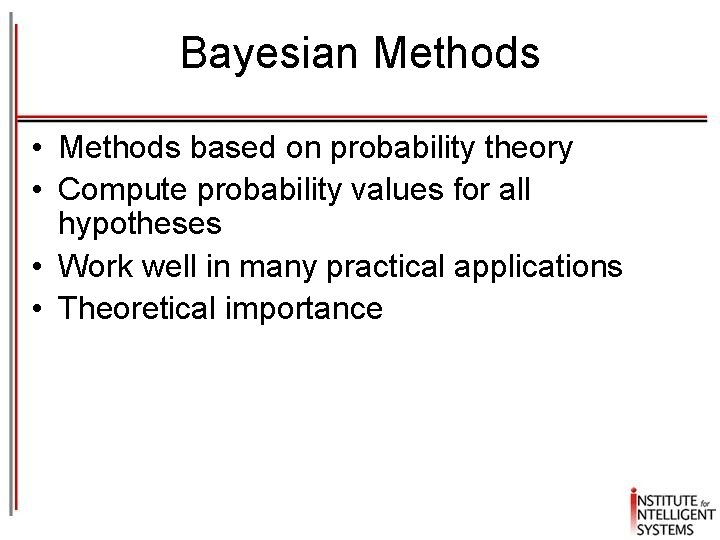Bayesian Methods • Methods based on probability theory • Compute probability values for all hypotheses • Work well in many practical applications • Theoretical importance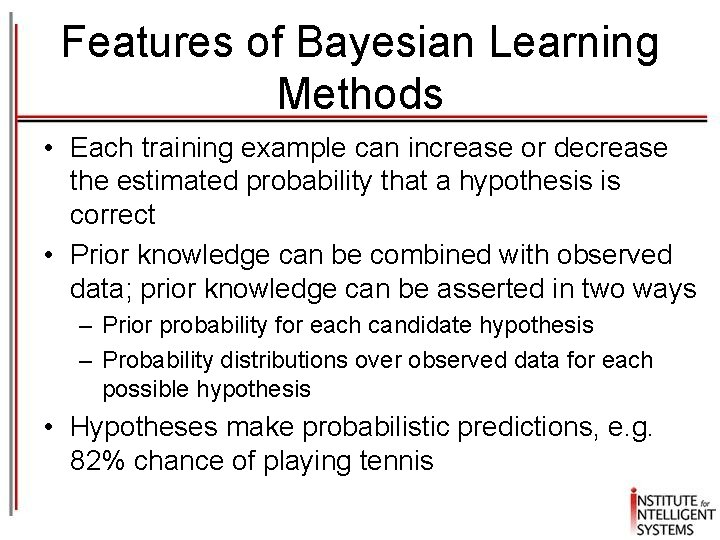Features of Bayesian Learning Methods • Each training example can increase or decrease the estimated probability that a hypothesis is correct • Prior knowledge can be combined with observed data; prior knowledge can be asserted in two ways – Prior probability for each candidate hypothesis – Probability distributions over observed data for each possible hypothesis • Hypotheses make probabilistic predictions, e. g. 82% chance of playing tennisFeatures of Bayesian Learning Methods • New instances may be handled using a combination of individual predictions from multiple hypotheses • Theoretical importance: provide a standard of optimal decision making against which other methods can be evaluated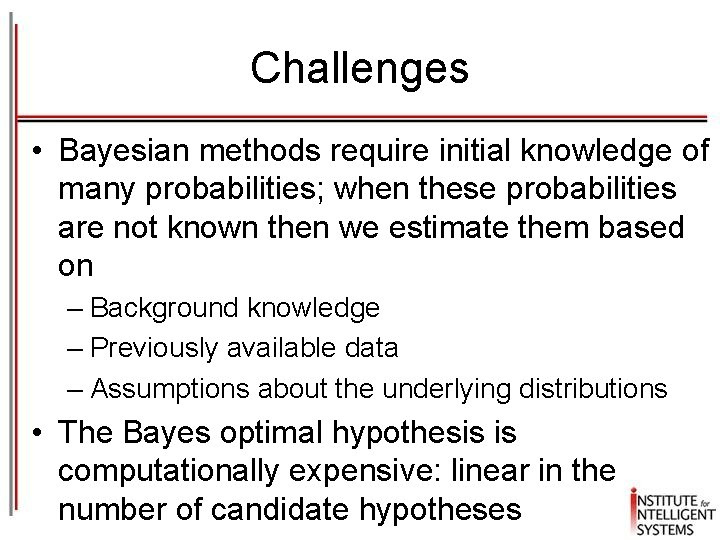Challenges • Bayesian methods require initial knowledge of many probabilities; when these probabilities are not known then we estimate them based on – Background knowledge – Previously available data – Assumptions about the underlying distributions • The Bayes optimal hypothesis is computationally expensive: linear in the number of candidate hypothesesBayesian Inference: Philosophical Perspective • Use evidence to change the degree of belief in a hypothesis – A two-face coin • Hypothesis: Heads – 50%; Tails – 50% – After 100 tosses you notice the head came up 95 times • Change the hypothesis based on the new evidenceAxioms of Probability Theory • All probabilities between 0 and 1 • True proposition has probability 1, false has probability 0 P(true) = 1 P(false) = 0 • The probability of disjunction is: A BConditional Probability • P(A | B) is the probability of A given B • Assumes that B is all and only information known • Defined by: A B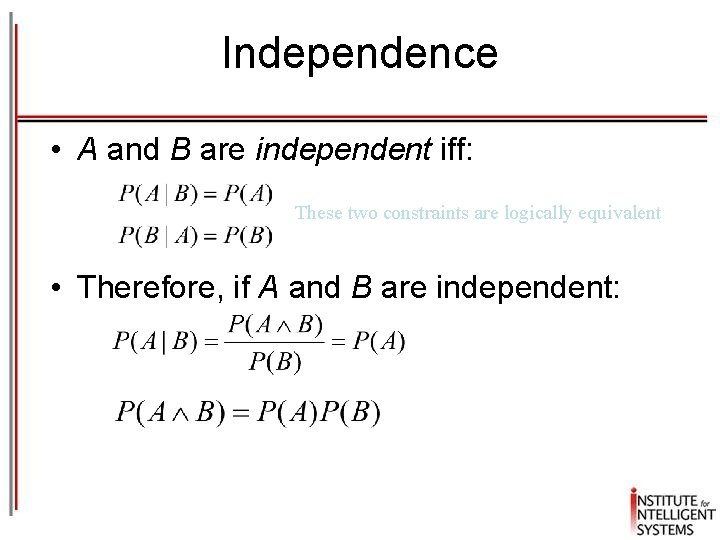Independence • A and B are independent iff: These two constraints are logically equivalent • Therefore, if A and B are independent:Bayes Theorem Simple proof from definition of conditional probability: (Def. cond. prob. ) QED: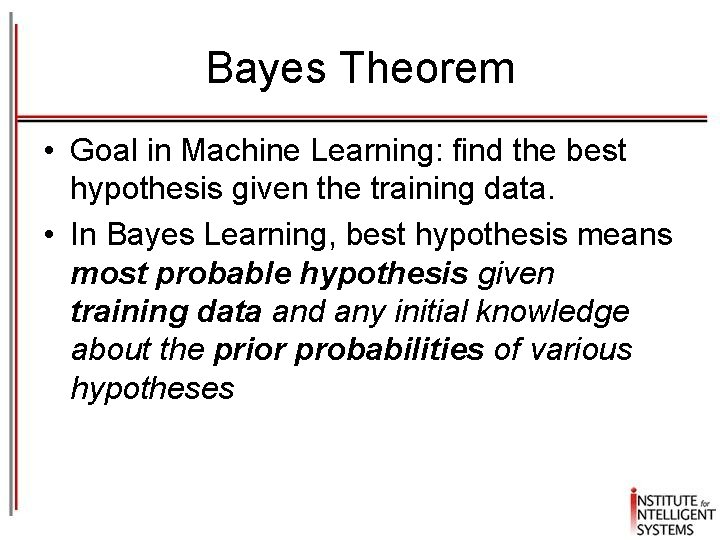Bayes Theorem • Goal in Machine Learning: find the best hypothesis given the training data. • In Bayes Learning, best hypothesis means most probable hypothesis given training data and any initial knowledge about the prior probabilities of various hypothesesBayes Theorem • Provides a way to calculate the probability of a hypothesis based on its prior probability, the probability of observing various data given the hypothesis, and the probability of observing the data itself. Likelihood Posterior Prior DataMaximum A Posteriori (MAP ) Hypothesis • The most probable hypothesis from the hypotheses space HMaximum Likelihood (ML) Hypothesis • When every hypothesis in H is equally probable: P(hi)=P(hj) for all hi and hj in H P(D|h) is called the likelihood of the data given h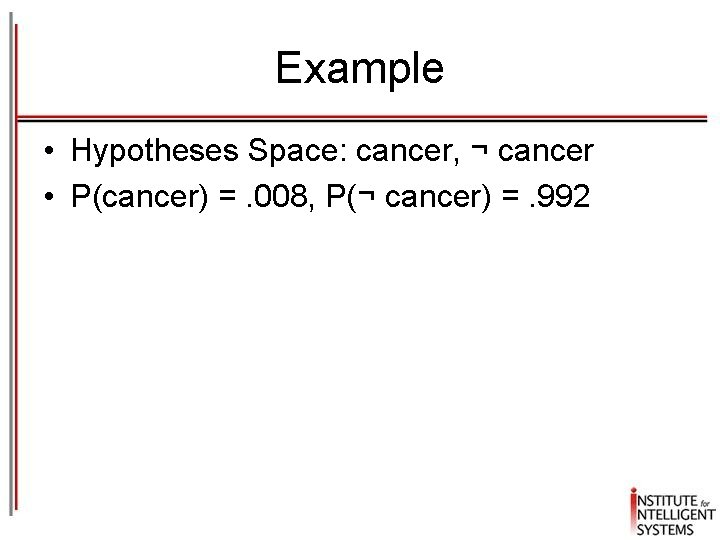Example • Hypotheses Space: cancer, ¬ cancer • P(cancer) =. 008, P(¬ cancer) =. 992Example • What if some symptoms are present? In the light of this new data, is/should our prior belief about someone having cancer changing/change? • New data: lab test – P(+|cancer) =. 98, P(-|cancer) =. 02 – P(+|¬cancer) =. 03, P(-|¬cancer) =. 97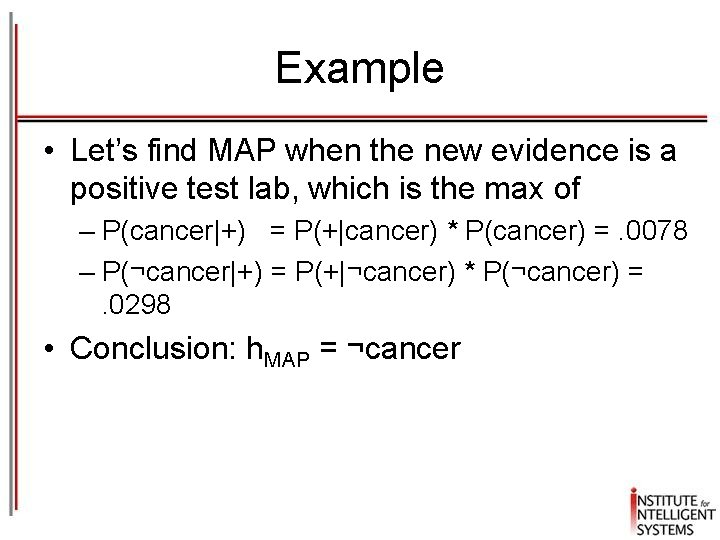Example • Let’s find MAP when the new evidence is a positive test lab, which is the max of – P(cancer|+) = P(+|cancer) * P(cancer) =. 0078 – P(¬cancer|+) = P(+|¬cancer) * P(¬cancer) = . 0298 • Conclusion: h. MAP = ¬cancerBayes Optimal Classifier •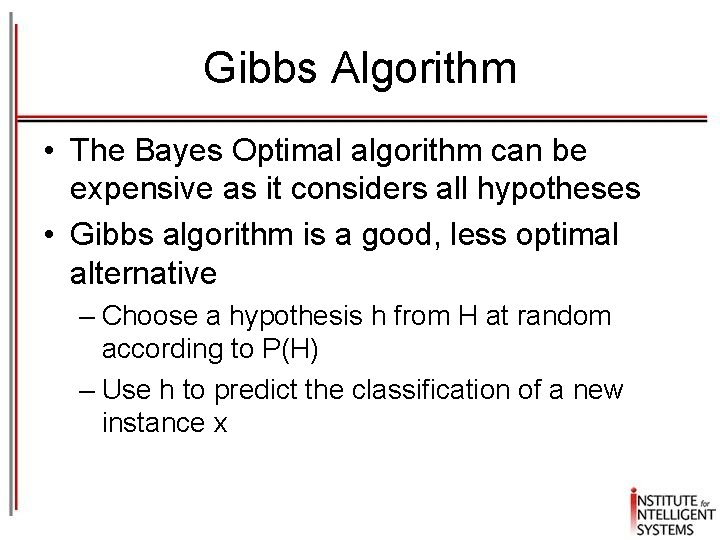Gibbs Algorithm • The Bayes Optimal algorithm can be expensive as it considers all hypotheses • Gibbs algorithm is a good, less optimal alternative – Choose a hypothesis h from H at random according to P(H) – Use h to predict the classification of a new instance x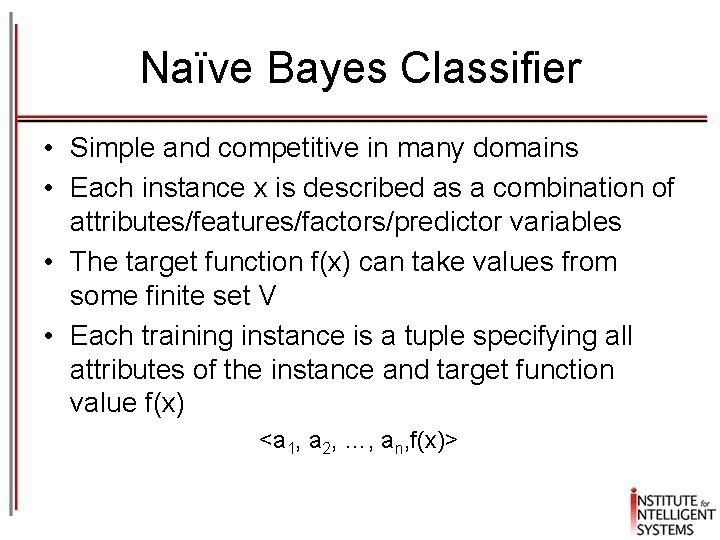Naïve Bayes Classifier • Simple and competitive in many domains • Each instance x is described as a combination of attributes/features/factors/predictor variables • The target function f(x) can take values from some finite set V • Each training instance is a tuple specifying all attributes of the instance and target function value f(x) <a 1, a 2, …, an, f(x)>Naïve Bayes MAP Naïve Assumption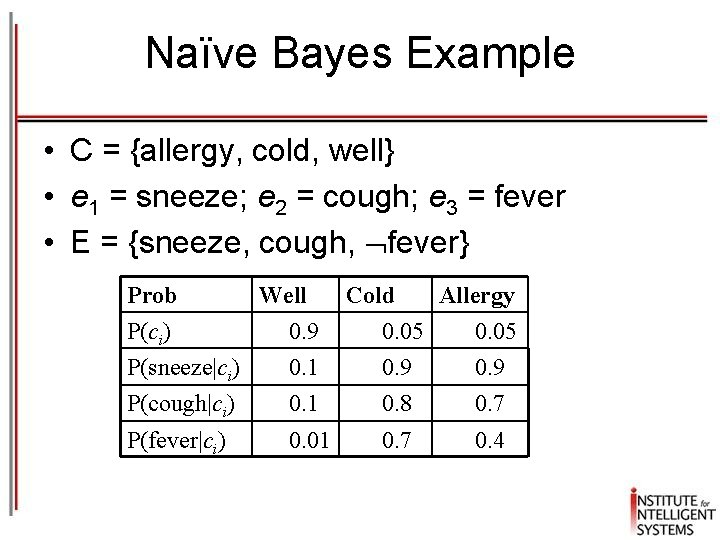Naïve Bayes Example • C = {allergy, cold, well} • e 1 = sneeze; e 2 = cough; e 3 = fever • E = {sneeze, cough, fever} Prob Well Cold Allergy P(ci) 0. 9 0. 05 P(sneeze|ci) 0. 1 0. 9 P(cough|ci) 0. 1 0. 8 0. 7 P(fever|ci) 0. 01 0. 7 0. 4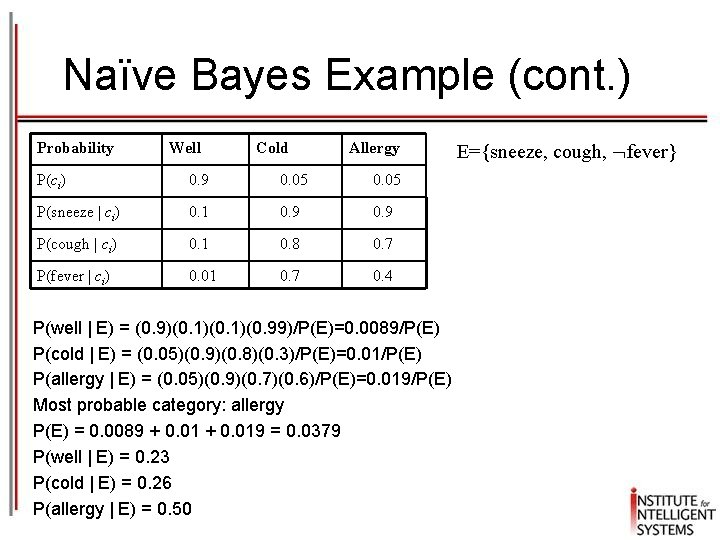Naïve Bayes Example (cont. ) Probability Well Cold Allergy P(ci) 0. 9 0. 05 P(sneeze | ci) 0. 1 0. 9 P(cough | ci) 0. 1 0. 8 0. 7 P(fever | ci) 0. 01 0. 7 0. 4 P(well | E) = (0. 9)(0. 1)(0. 99)/P(E)=0. 0089/P(E) P(cold | E) = (0. 05)(0. 9)(0. 8)(0. 3)/P(E)=0. 01/P(E) P(allergy | E) = (0. 05)(0. 9)(0. 7)(0. 6)/P(E)=0. 019/P(E) Most probable category: allergy P(E) = 0. 0089 + 0. 019 = 0. 0379 P(well | E) = 0. 23 P(cold | E) = 0. 26 P(allergy | E) = 0. 50 E={sneeze, cough, fever}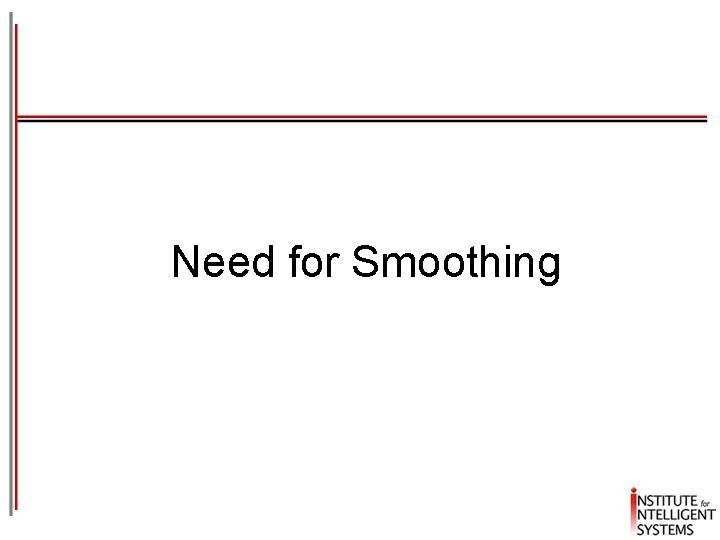Need for SmoothingEstimating Probabilities • Normally, probabilities are estimated based on observed frequencies in the training data • If D contains ni examples in category ci, and nij of these ni examples contains attribute aj, then: • However, estimating such probabilities from small training sets is error-prone • If due only to chance, a rare feature, ak, is always false in the training data, ci : P(ak | ci) = 0 • If ak then occurs in a test example, x, the result is that ci: P(x|ci) = 0 and ci: P(ci | x) = 0Smoothing • To account for estimation from small samples, probability estimates are adjusted or smoothed • Laplace smoothing using an m-estimate assumes that each feature is given a prior probability, p, that is assumed to have been previously observed in a “virtual” sample of size m • For binary features, p is simply assumed to be 0. 5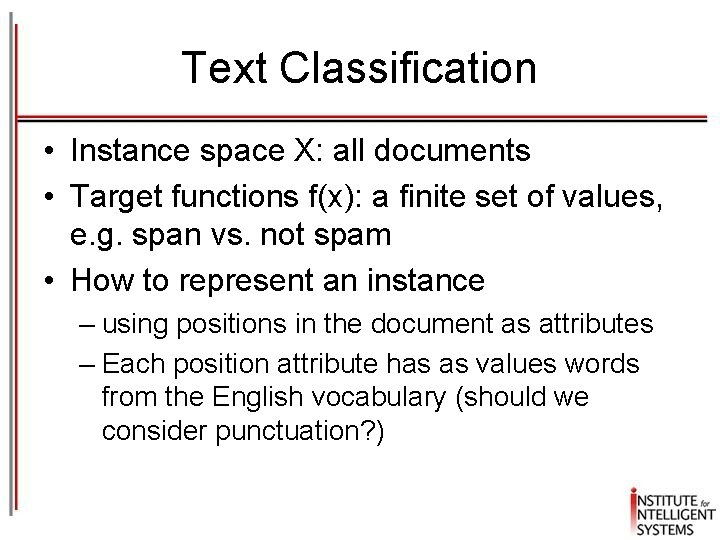Text Classification • Instance space X: all documents • Target functions f(x): a finite set of values, e. g. span vs. not spam • How to represent an instance – using positions in the document as attributes – Each position attribute has as values words from the English vocabulary (should we consider punctuation? )Using Naive Bayes Classifiers to Classify Text: Basic method • Attributes are text positions, values are words • Still too many possibilities • Assume that classification is independent of the positions of the words – Use same parameters for each position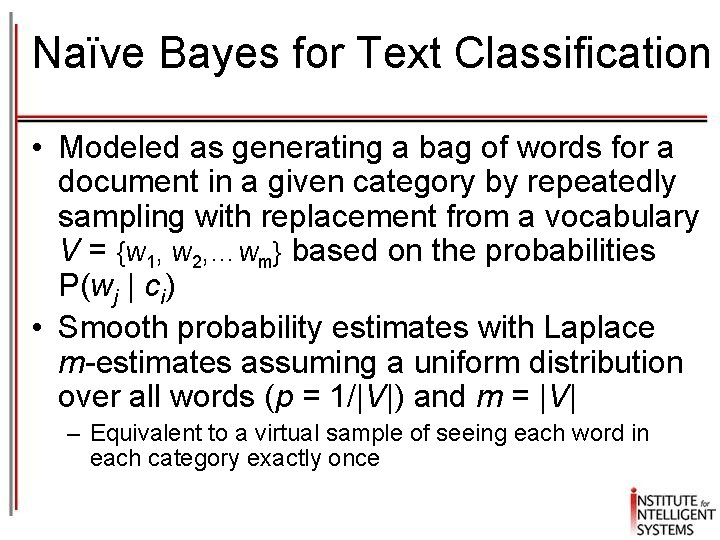Naïve Bayes for Text Classification • Modeled as generating a bag of words for a document in a given category by repeatedly sampling with replacement from a vocabulary V = {w 1, w 2, …wm} based on the probabilities P(wj | ci) • Smooth probability estimates with Laplace m-estimates assuming a uniform distribution over all words (p = 1/|V|) and m = |V| – Equivalent to a virtual sample of seeing each word in each category exactly onceText Naïve Bayes Algorithm (Train) Let V be the vocabulary of all words in the documents in D For each category ci C Let Di be the subset of documents in D in category ci P(ci) = |Di| / |D| Let Ti be the concatenation of all the documents in Di Let ni be the total number of word occurrences in Ti For each word wj V Let nij be the number of occurrences of wj in Ti Let P(wi | ci) = (nij + 1) / (ni + |V|)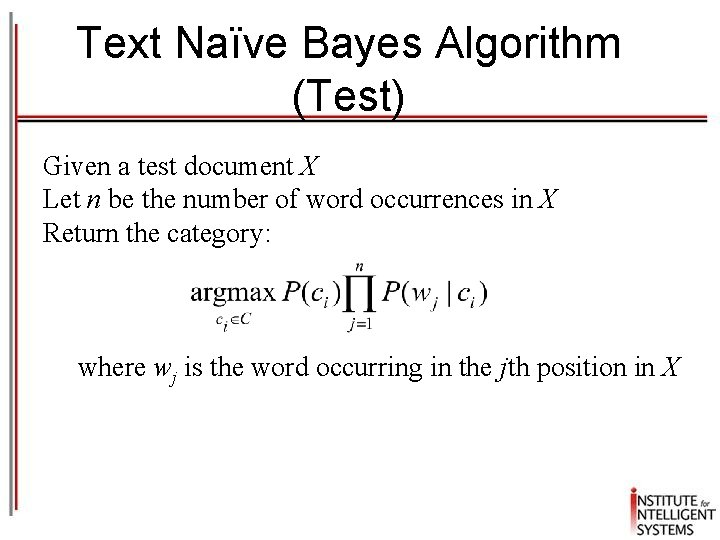Text Naïve Bayes Algorithm (Test) Given a test document X Let n be the number of word occurrences in X Return the category: where wj is the word occurring in the jth position in X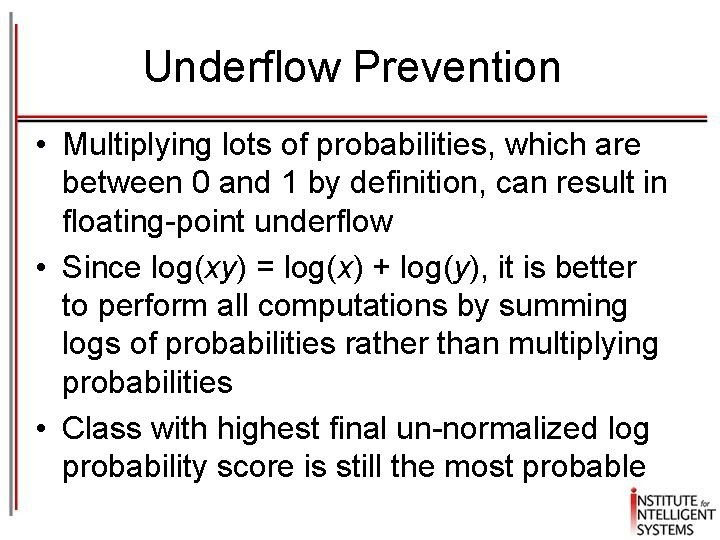Underflow Prevention • Multiplying lots of probabilities, which are between 0 and 1 by definition, can result in floating-point underflow • Since log(xy) = log(x) + log(y), it is better to perform all computations by summing logs of probabilities rather than multiplying probabilities • Class with highest final un-normalized log probability score is still the most probableNaïve Bayes Posterior Probabilities • Classification results of naïve Bayes (the class with maximum posterior probability) are usually fairly accurate • However, due to the inadequacy of the conditional independence assumption, the actual posteriorprobability numerical estimates are not – Output probabilities are generally very close to 0 or 1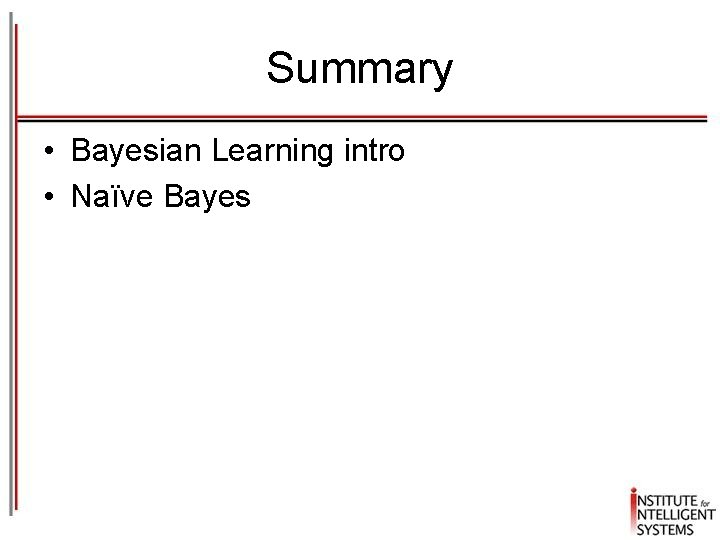Summary • Bayesian Learning intro • Naïve Bayes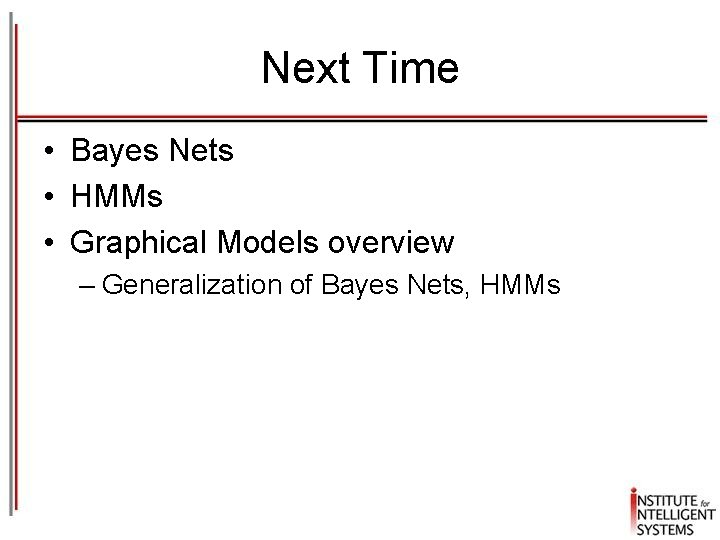Next Time • Bayes Nets • HMMs • Graphical Models overview – Generalization of Bayes Nets, HMMs